# Decagon

Go back to  'Solid Shapes'

 1 Introduction To Decagons 2 What is a Decagon? 3 Types of Decagons 4 Challenging Questions on Decagons 5 Properties of Decagons 6 Important Notes on Decagons 7 Solved Examples on Decagons 8 Practice Questions on Decagons 9 Maths Olympiad Sample Papers 10 Frequently Asked Questions (FAQs)

We at Cuemath believe that Math is a life skill. Our Math Experts focus on the “Why” behind the “What.” Students can explore from a huge range of interactive worksheets, visuals, simulations, practice tests, and more to understand a concept in depth.

Book a FREE trial class today! and experience Cuemath's LIVE Online Class with your child.

## Introduction To Decagons

A decagon is a ten-sided polygon with ten vertices and ten angles.

### What are Polygons?

In geometry, a polygon is a flat, plane shape, usually two-dimensional consisting of straight and non-intersecting lines or sides.

These shapes have three or more sides and thus, the name "polygon" is given to them as "poly" means many in Greek while "gon" refers to the shape.

Thus, a polygon is a shape with many sides.

 Sides Polygon 3 Triangle 4 Quadrilateral 5 Pentagon 6 Hexagon 7 Heptagon 8 Octagon 9 Nonagon 10 Decagon

A regular polygon has all sides and all angles congruent.

An irregular polygon has unequal sides and angles.

## What is a Decagon?

You have already studied shapes and polygons in detail.

The word “decagon” dates back to the 16th century.

It is derived from the Latin word "decagonum," wherein "deca" means ten and "gonum" means angles.

Hence, the word "decagon" refers to ten angles.

The shape of a Decagon: A decagon is a ten-sided polygon with ten vertices and ten angles.

Here are some examples of a decagon.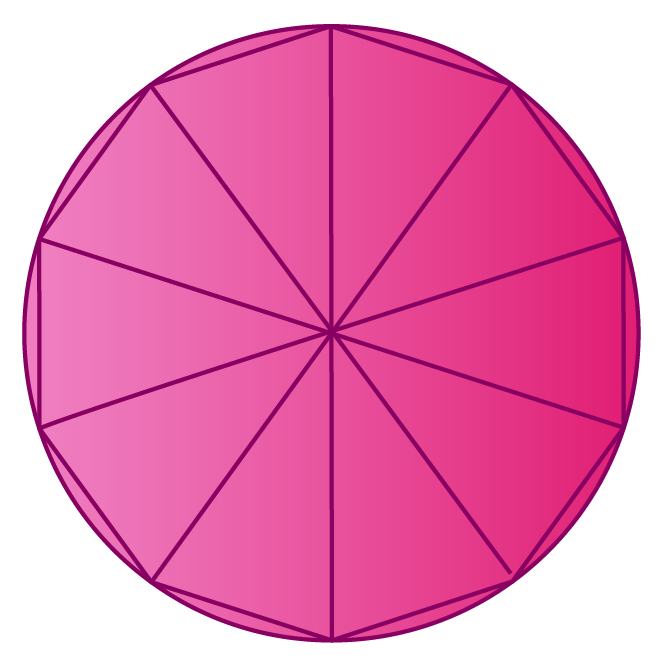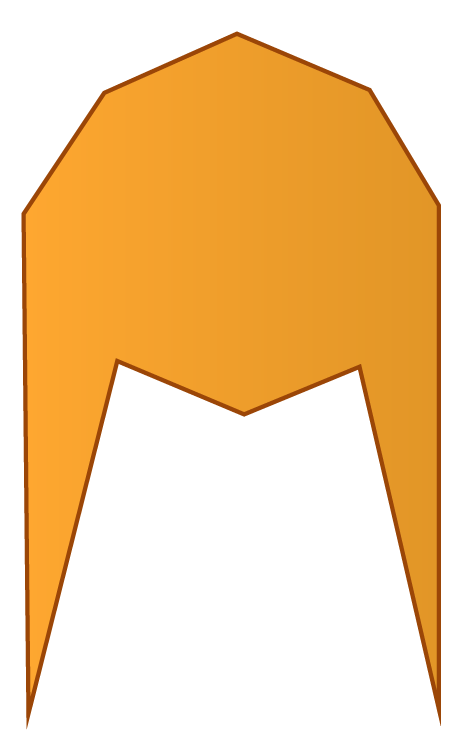Examples of Decagon

Thus, a decagon shape can be defined as a polygon having ten sides, ten interior angles and ten vertices.

Based on the sides of a decagon, they are broadly classified into regular decagons and irregular decagons.

A regular decagon has 35 diagonals and 8 triangles.

## Types of Decagons

Decagons can be categorized as:

• Regular Decagons
• Irregular Decagons

### Regular Decagon

1. A regular decagon is a decagon with all sides equal in length and all the angles equal in measure.
2. In a regular decagon, the interior angles add up to $$1440\ ^\circ$$ and the exterior angles add up to $$360\ ^\circ$$
3. The sides and angles in a regular decagon are congruent.

### Irregular Decagon

1. An irregular decagon is a type of decagon with unequal sides and angles.
2. There are not congruent.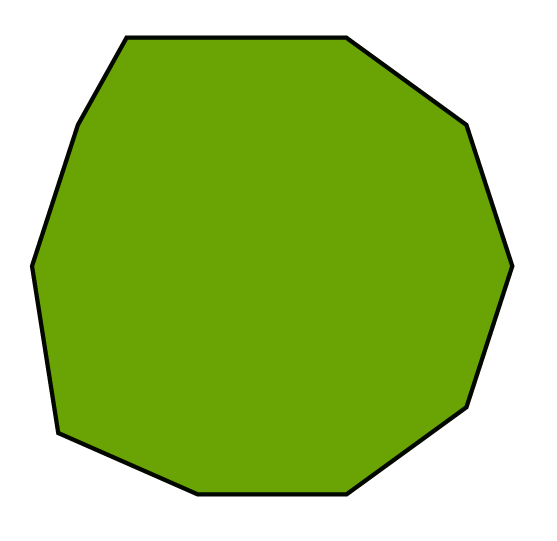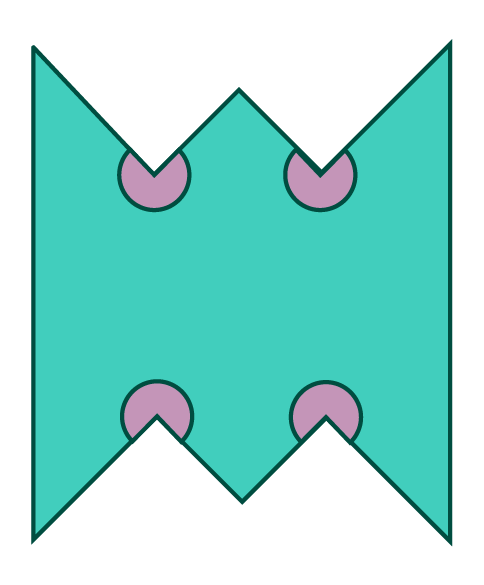### Convex and Concave Decagons

Like any other polygon, decagons also can be convex and concave.

Convex Decagon:

1. Convex decagons bulge outward.
2. None of their interior angles is greater than $$180\ ^\circ$$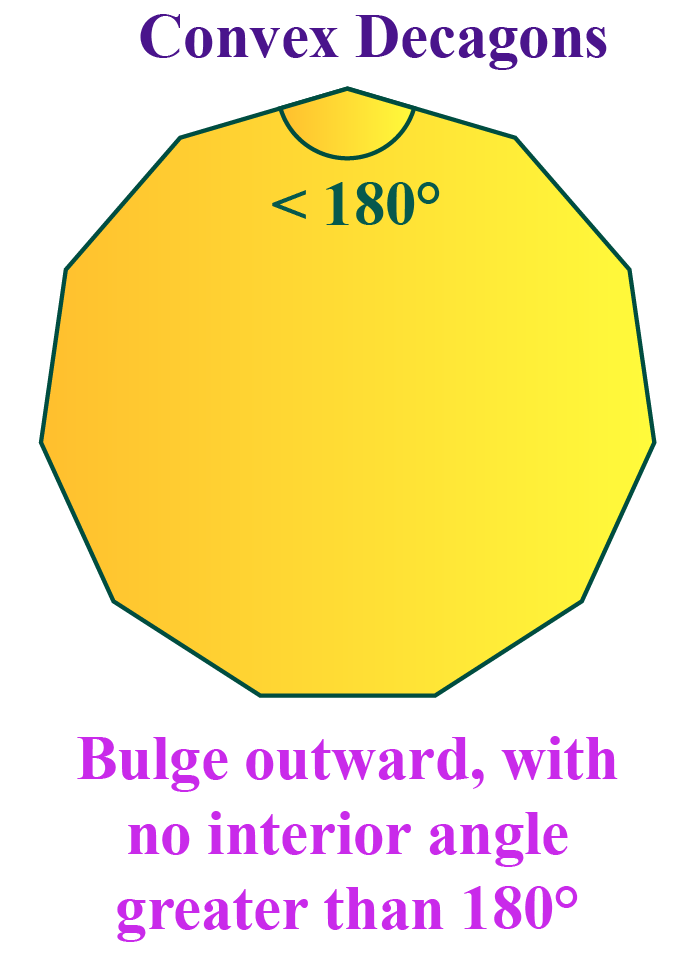Concave decagon:

1. Concave decagons have indentations (a deep recess)
2. The interior angles are greater than $$180\ ^\circ$$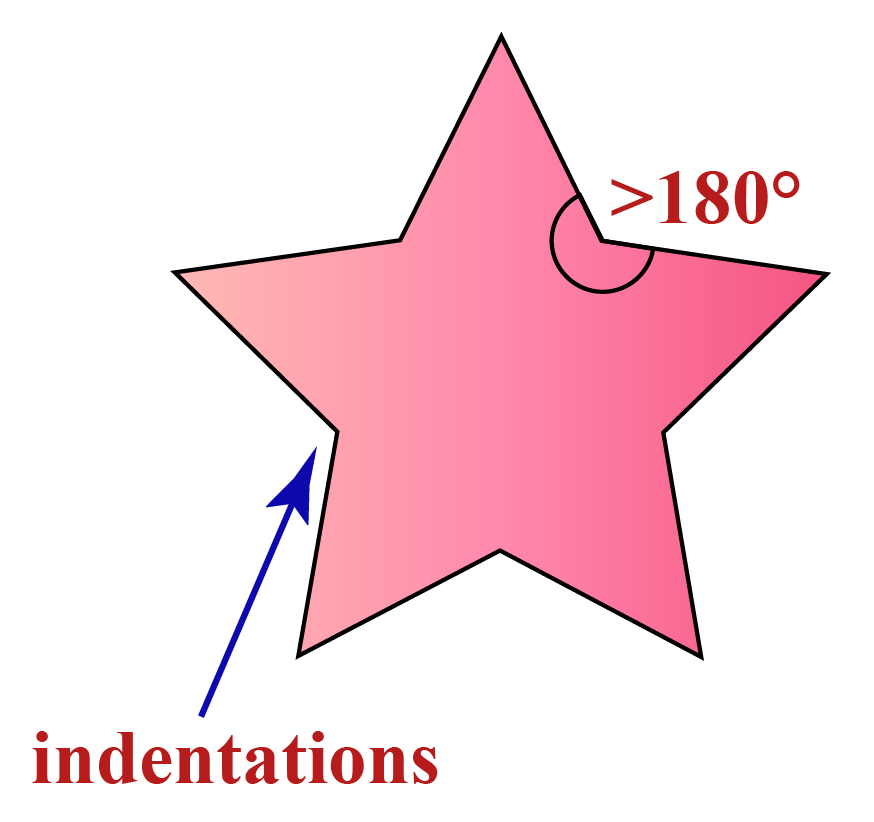### Simple and Complex Decagons

Simple Decagons: They refer to decagons with no sides crossing themselves.

They follow all of the above said regular decagon rules.

Complex Decagon: They refer to decagons that are self-intersecting and have additional interior spaces.

They do not strictly follow any prescribed rules of decagons regarding their interior angles and their sums.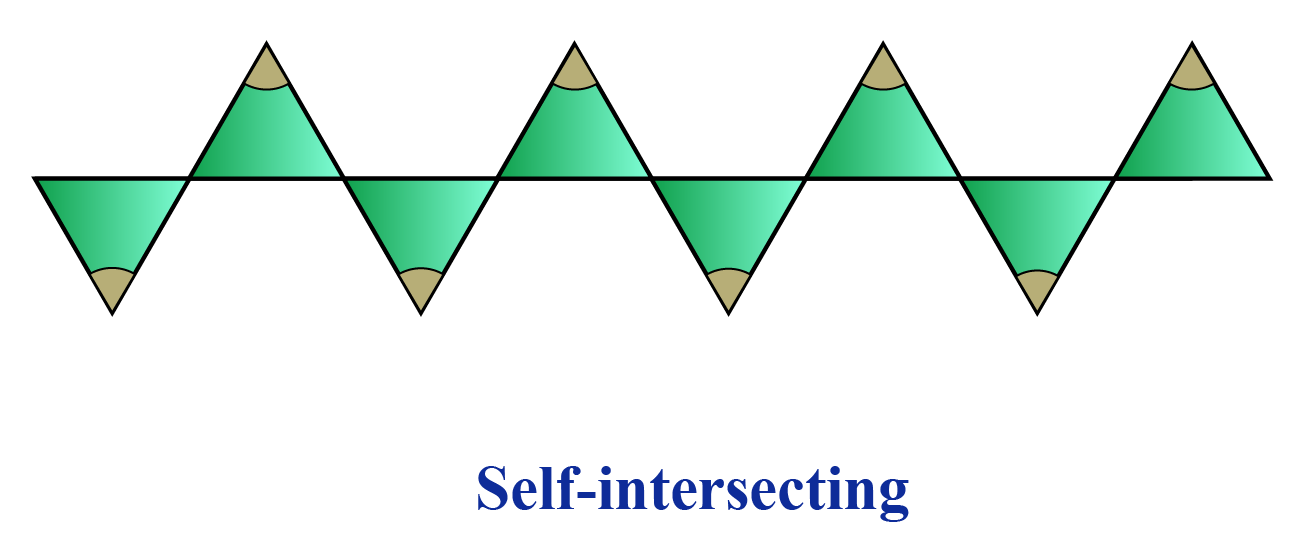Challenging Questions
1. What is the sum of the number of sides, vertices, and angles in an irregular decagon?
a) 10               b) 20                  c) 30               d) 40
2. What is the number of triangles in a decagon?

## Properties of Decagons

Decagons can be commonly seen in various things in our surroundings.

We can observe them in coasters, umbrellas, coins, watches, drums, cutlery, and so on.

A decagon is an interesting form of a polygon and is widely used in creating wall art.

Let us now learn a few properties of decagons.

Some of the important properties of regular decagons are listed here.

• The sum of the interior angles is $$1440\ ^\circ$$
• The measure of the central angle is $$36\ ^\circ$$
• They are 35 diagonals in a decagon.
• They are 8 triangles in a decagon.

### Sum of the Interior Angles

To find the sum of the interior angles of a decagon, first, divide it into triangles.

There are eight triangles in a regular decagon.

We know that the sum of the angles in each triangle is $$180\ ^\circ$$

Thus,

\begin{align} 180\ ^\circ \times 8 = 1440 ^\circ \end{align}

Therefore, the sum of all the interior angles of a decagon is $$1440\ ^\circ$$

So, how do we get the measure of one interior angle?

We know that the number of sides of a decagon is 10

Hence, we divide the total sum of the interior angles by 10

\begin{align} 1440\ ^\circ \div 10 = 144 ^\circ\end{align}

Thus, one interior angle of decagon shape is $$144 ^\circ$$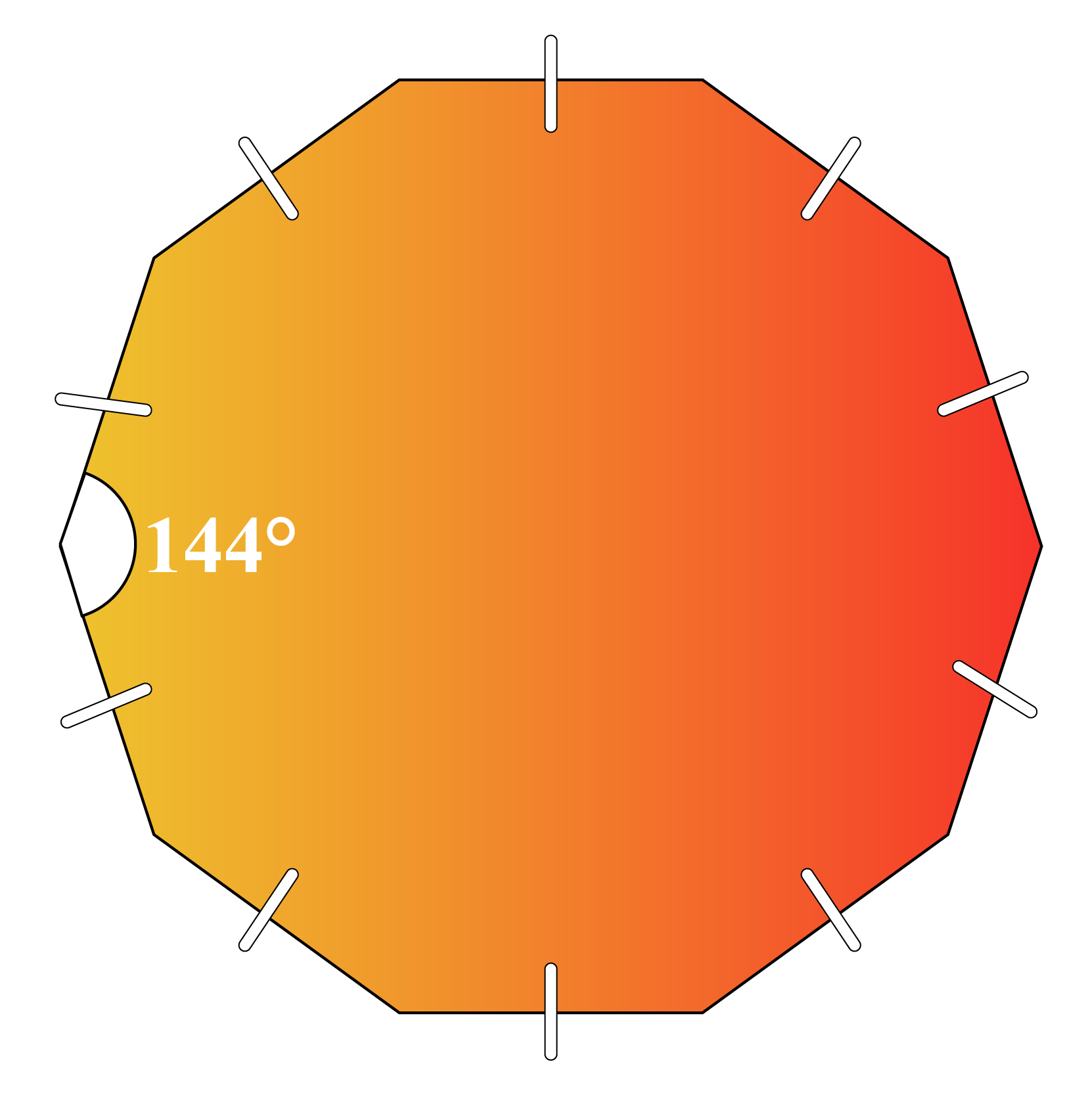Each interior angle is $$144\ ^\circ$$

And, the sum of all the interior angles of a decagon is $$1440\ ^\circ$$

Let us explore this concept with the simulation below.

You can move any of the vertices in the figure and then observe the sum of its interior angles.

The result will remain the same.

### The Measure of the Central Angles of a Regular Decagon

To find the measure of the central angle of a regular decagon, we need to draw a circle in the middle.

A circle is $$360\ ^\circ$$

Divide this by ten, because a decagon has 10 sides.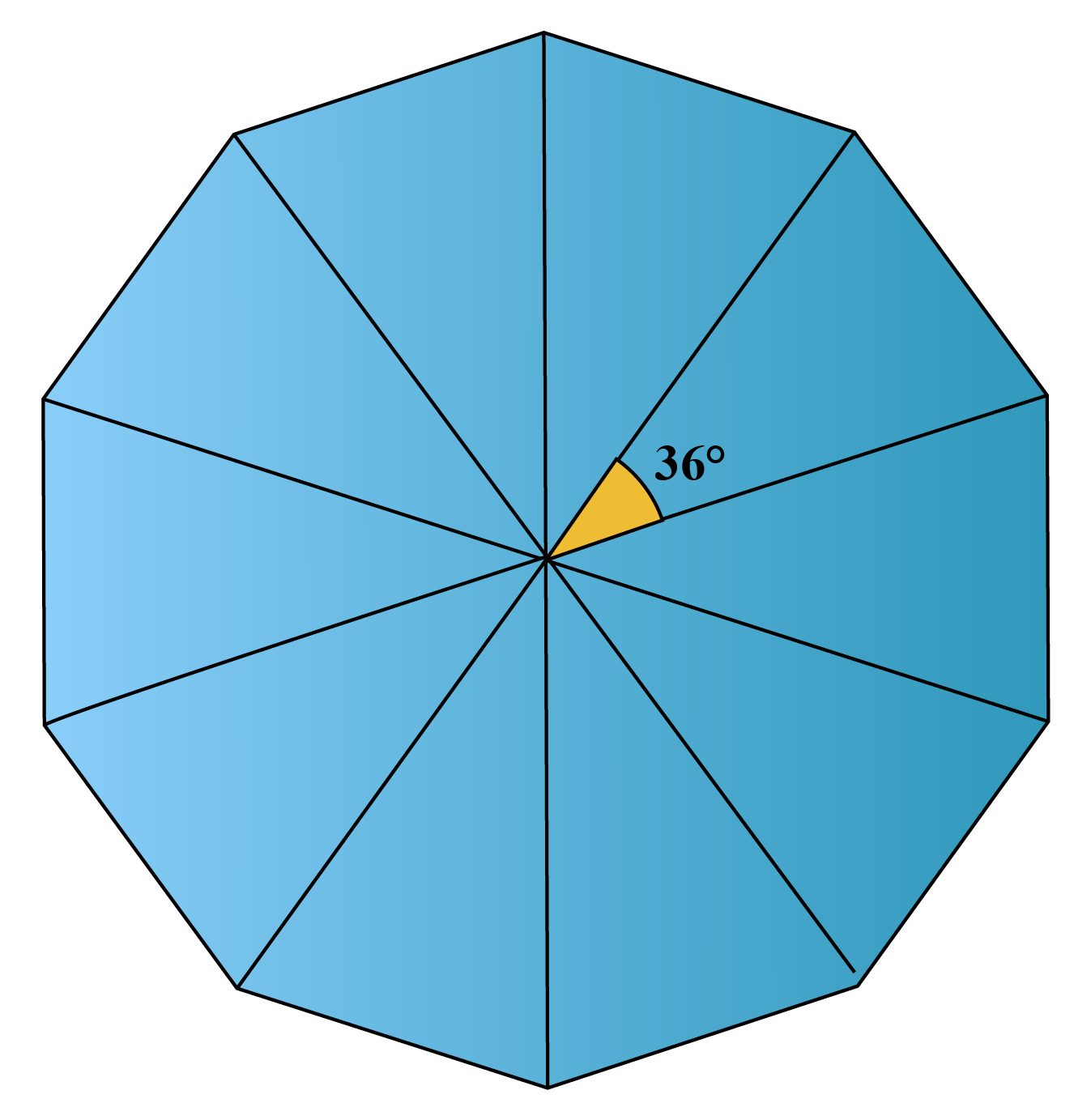\begin{align} 360\ ^\circ \div 10 = 36 ^\circ \end{align}

Thus, the measure of the central angle of a regular decagon is $$36\ ^\circ$$

 The central angle of a regular decagon is $$36\ ^\circ$$

### Decagon Diagonals

A diagonal is a line that can be drawn from one vertice to another.

The number of Diagonals of a polygon is calculated by:

\begin{align} \frac{n(n-3)}{2} \end{align}

In Decagon, $$n$$ is the number of sides which is equal to 10

$n = 10$

$\frac{10(10-3)}{2}$

$35$

Thus, the number of diagonals in a decagon is 35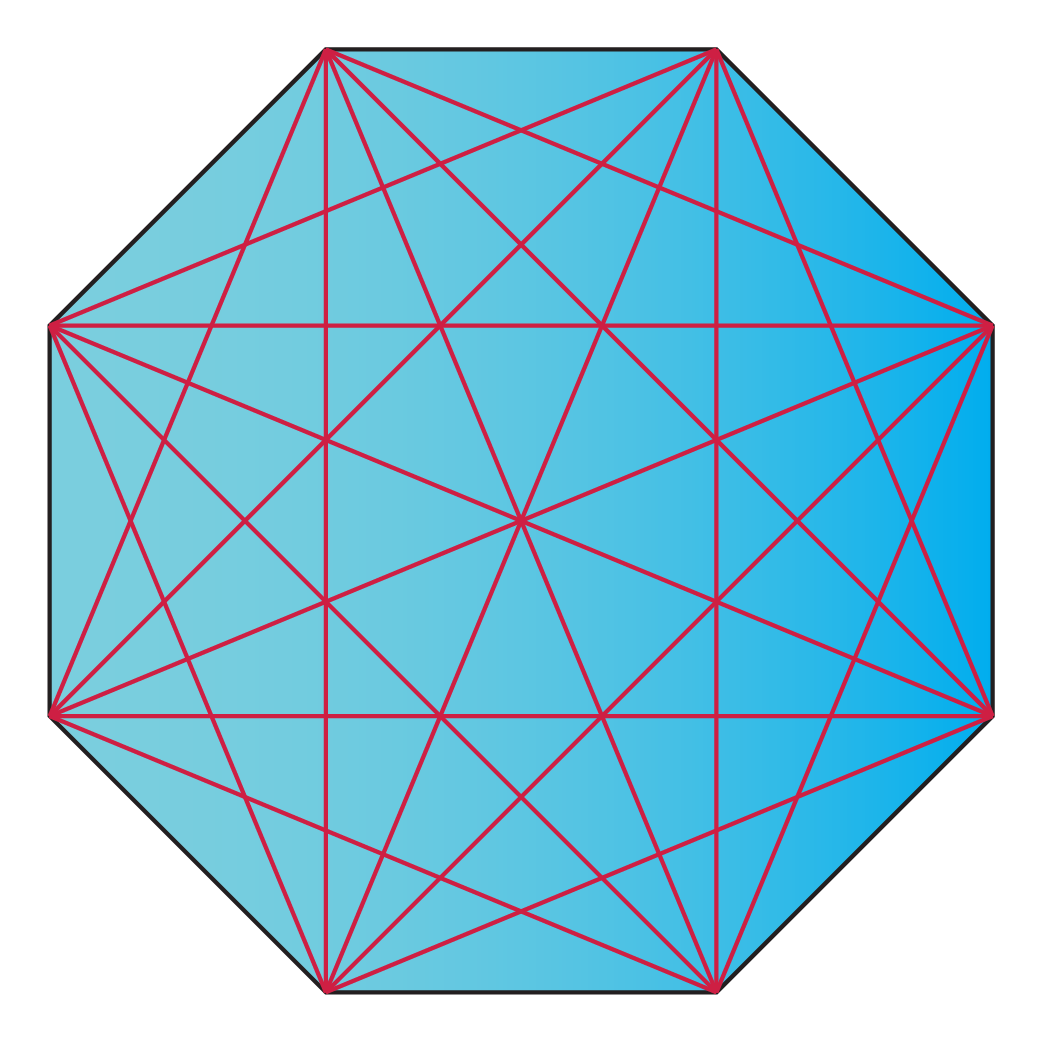35 diagonals in a regular decagon

Explore above with the above simulation by checking the box beside each vertex. It shows the possible diagonal from that vertex.In total after all boxes are checked, we get 35 diagonals.

 A decagon has 35 diagonals

Help your child score higher with Cuemath’s proprietary FREE Diagnostic Test. Get access to detailed reports, customized learning plans, and a FREE counseling session. Attempt the test now.

## Solved Examples on Decagons

 Example 1

Identify three decagon shapes that we use in our day-to-day activities.

Which of the household items shown below have the shape of a decagon?

Drag and check with the decagon template provided.

Solution:

Drag the image to the template provided and check if they have the shape of a decagon.

 $$\therefore$$ The watch shown above has the shape of a decagon.
 Example 2

Jenifer's hobby is collecting coins from various countries.

She found a new coin to add to her collection.

Can you guess whether this coin resembles a decagon shape or not?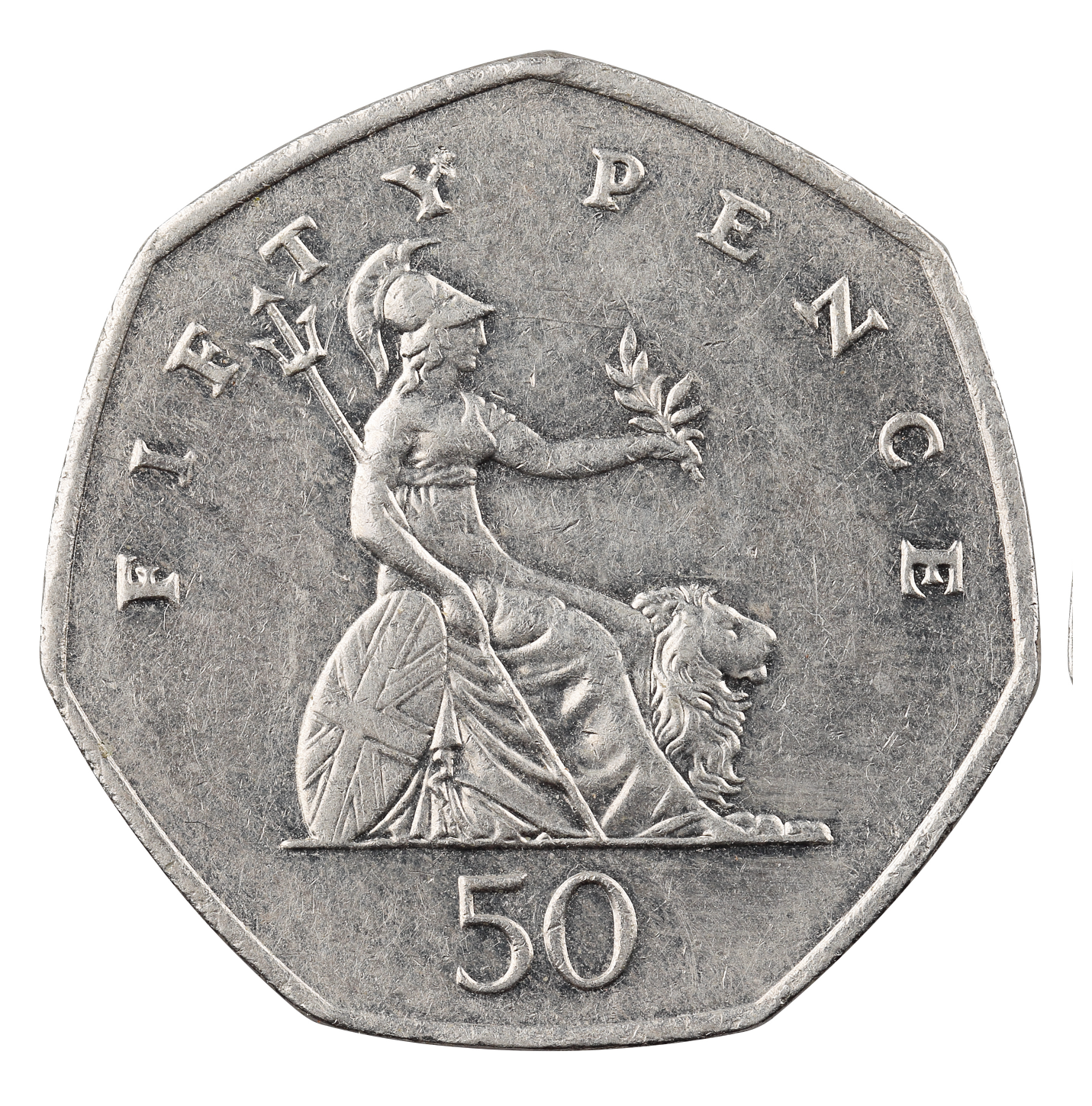A. YES
B. NO
Solution:

Decagon has ten sides and ten angles.

 $$\therefore$$ NO, Coin is not in a decagon shape.
 Example 3

Identify the irregular decagon from the choices below.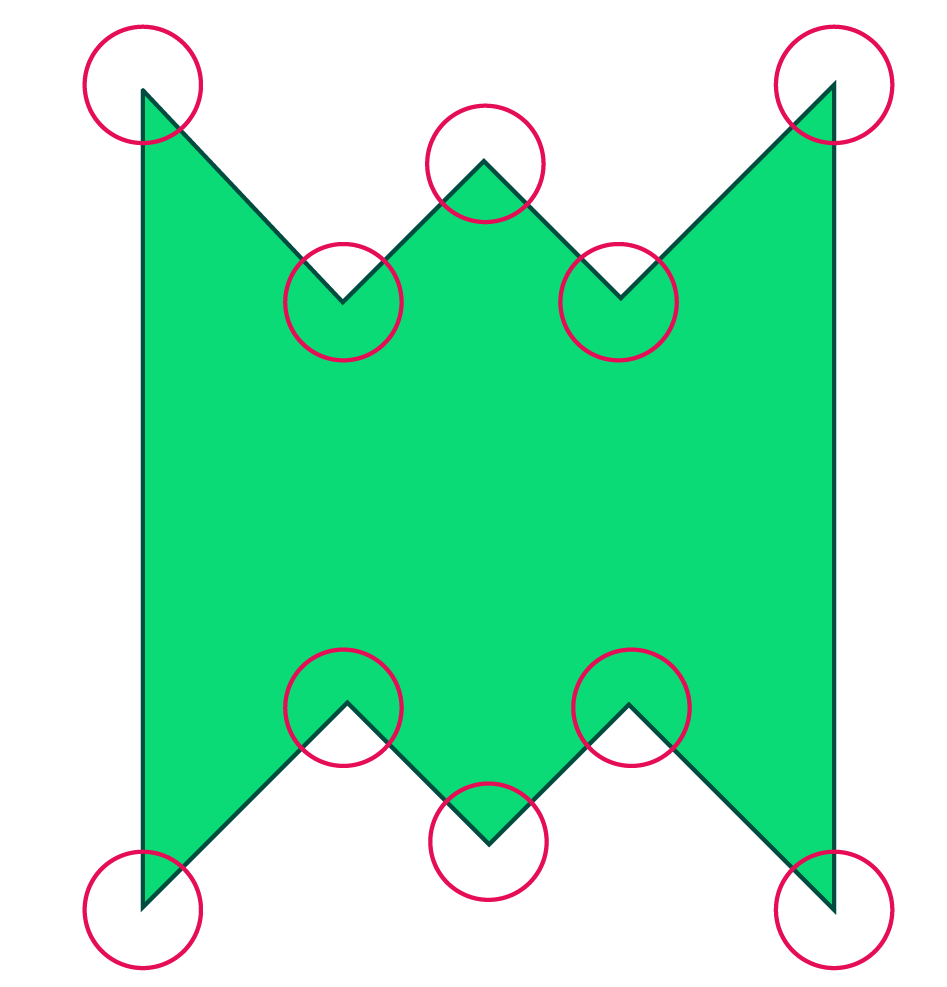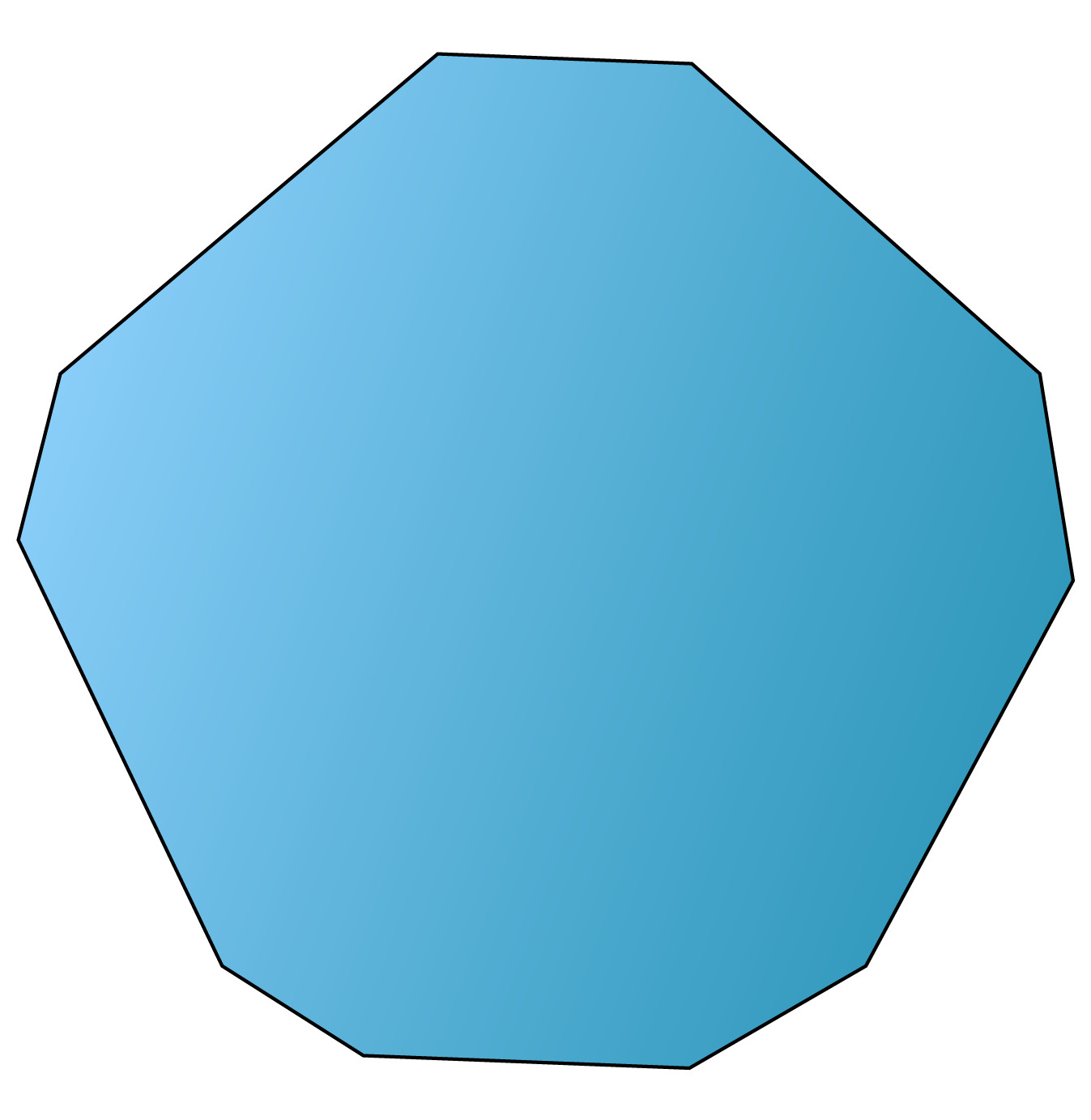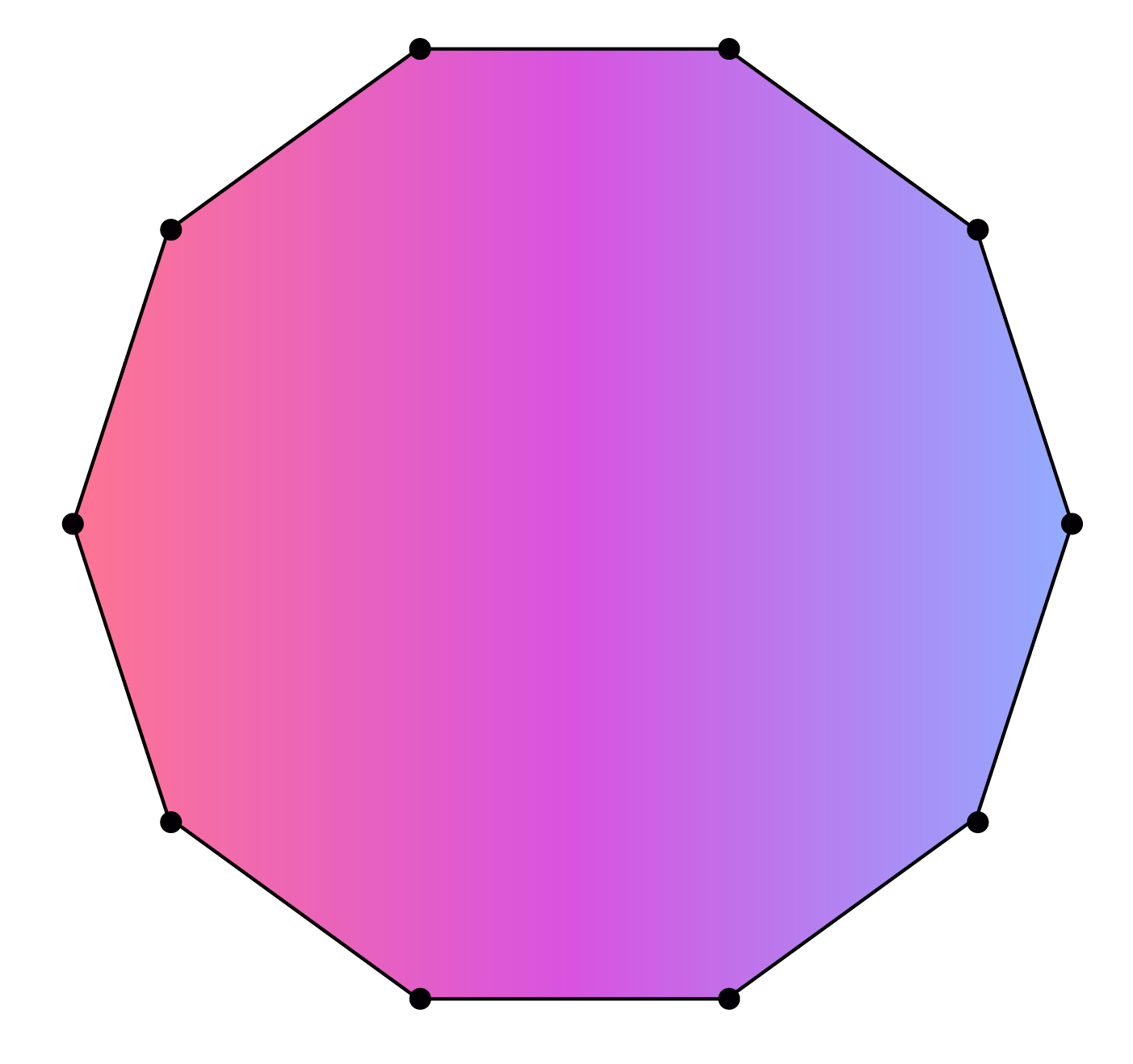Solution:

a) Irregular as its angles are not equal.

b) Irregular as its sides are not equal.

c) Regular as it has equal sides and equal angles.

 $$\therefore$$ (a) and (b) are irregular decagons.
 Example 4

How many triangles can be formed by joining the vertices of a decagon?

Solution:

In a decagon, by joining one vertex to the remaining vertices, you can have 8 triangles.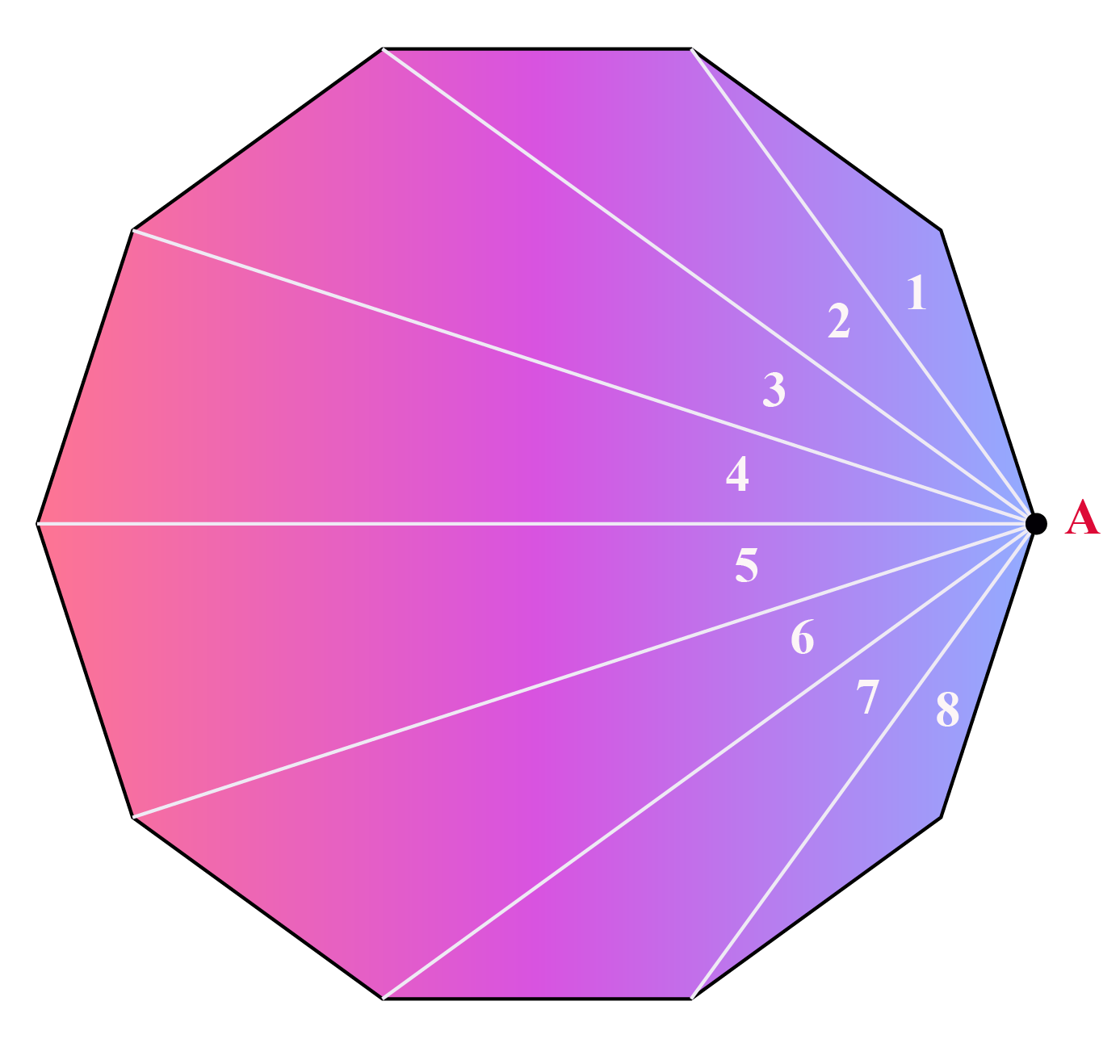Similarly, If you are considering all the vertices independently you will have a total of $$8\times 10 = 80$$ triangles.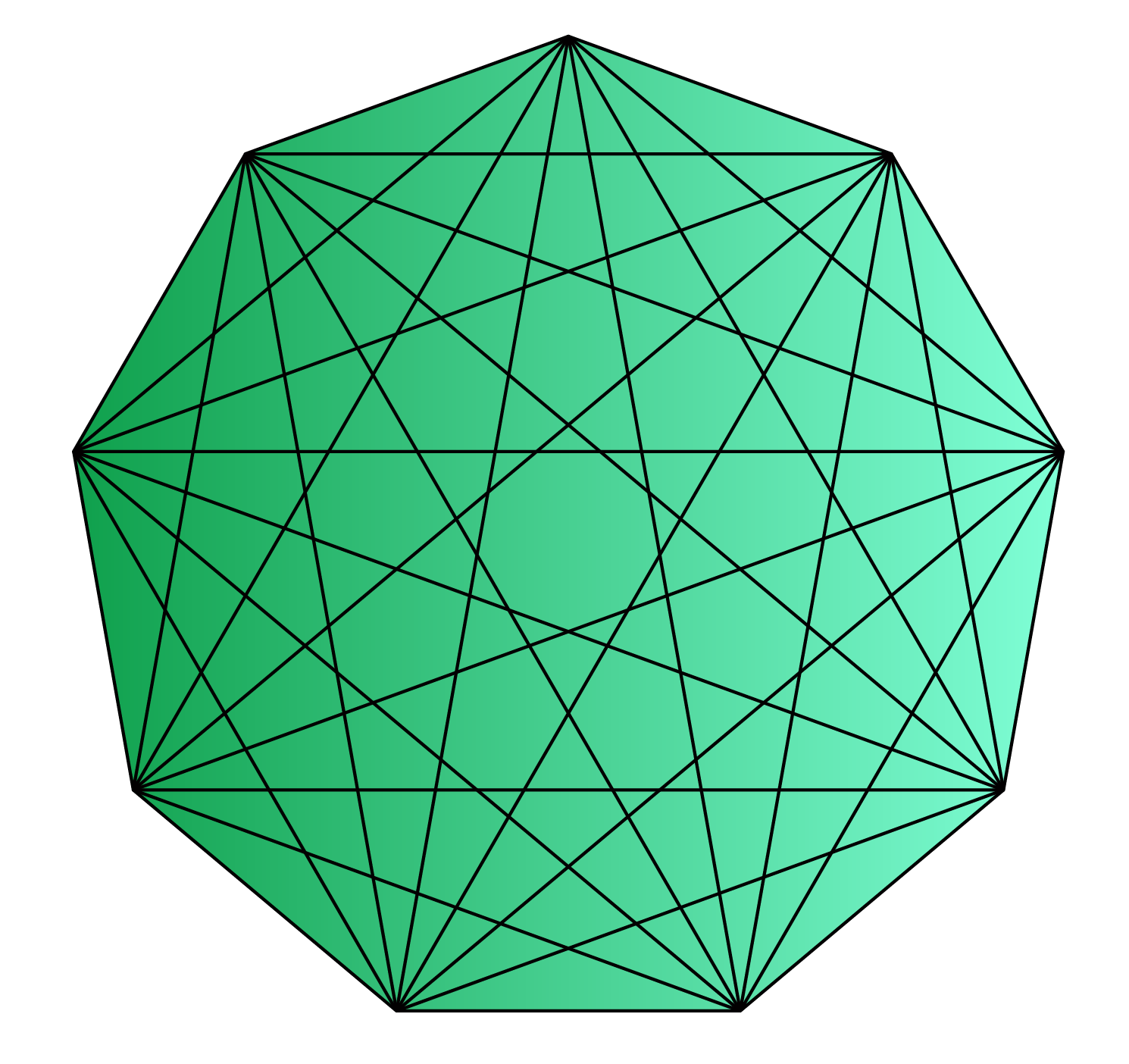$$\therefore$$ 8 traingles from one vertex and 80 triangles from 10 vertices.
 Example 5

Which decagon shape does this starfish have?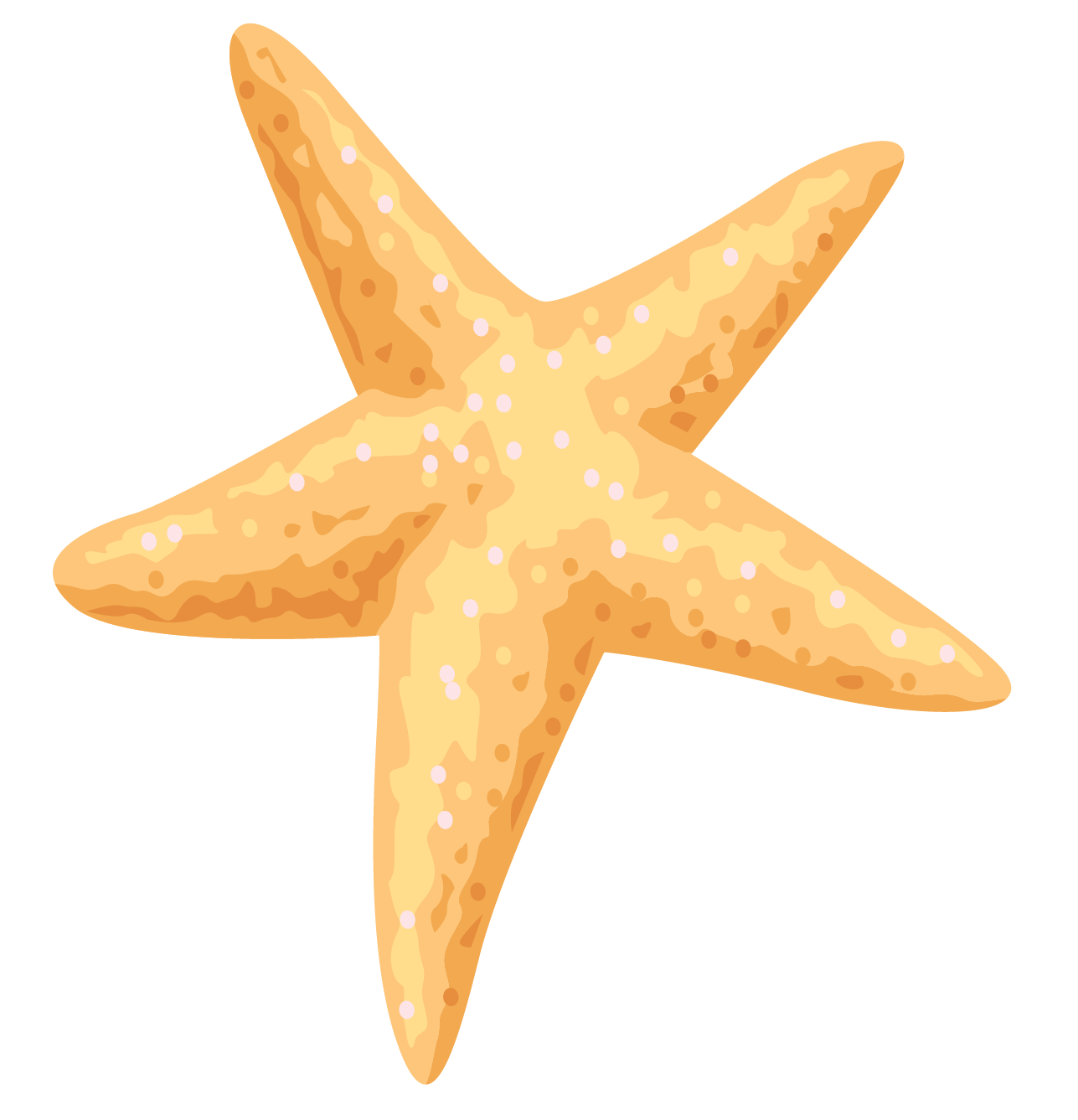a) Concave Decagon

b) Convex Decagon

Solution:

a) Concave Decagon: Its sides have indentations and its interior angles are greater than $$180\ ^\circ$$

 $$\therefore$$ The starfish is a concave decagon.

More Important Topics
Numbers
Algebra
Geometry
Measurement
Money
Data
Trigonometry
Calculus